## 2.1.2 Examples of Discrete Planning

Example 2..1 (Moving on a 2D Grid)   Suppose that a robot moves on a grid in which each grid point has integer coordinates of the form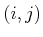. The robot takes discrete steps in one of four directions (up, down, left, right), each of which increments or decrements one coordinate. The motions and corresponding state transition graph are shown in Figure 2.1, which can be imagined as stepping from tile to tile on an infinite tile floor.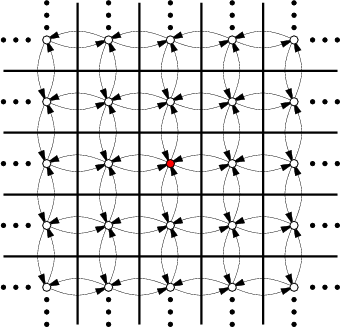This will be expressed using Formulation 2.1. Letbe the set of all integer pairs of the form, in which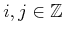(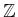denotes the set of all integers). Let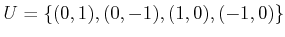. Let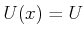for all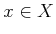. The state transition equation is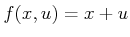, in whichand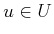are treated as two-dimensional vectors for the purpose of addition. For example, if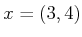and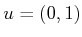, then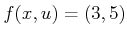. Suppose for convenience that the initial state is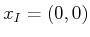. Many interesting goal sets are possible. Suppose, for example, that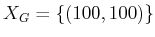. It is easy to find a sequence of actions that transforms the state from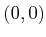to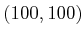.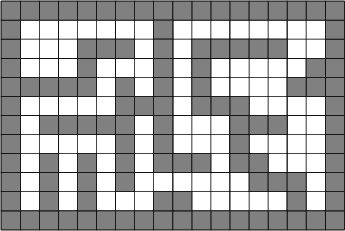The problem can be made more interesting by shading in some of the square tiles to represent obstacles that the robot must avoid, as shown in Figure 2.2. In this case, any tile that is shaded has its corresponding vertex and associated edges deleted from the state transition graph. An outer boundary can be made to fence in a bounded region so thatbecomes finite. Very complicated labyrinths can be constructed.Example 2..2 (Rubik's Cube Puzzle)   Many puzzles can be expressed as discrete planning problems. For example, the Rubik's cube is a puzzle that looks like an array oflittle cubes, which together form a larger cube as shown in Figure 1.1a (Section 1.2). Each face of the larger cube is painted one of six colors. An action may be applied to the cube by rotating a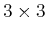sheet of cubes by 90 degrees. After applying many actions to the Rubik's cube, each face will generally be a jumble of colors. The state space is the set of configurations for the cube (the orientation of the entire cube is irrelevant). For each state there are 12 possible actions. For some arbitrarily chosen configuration of the Rubik's cube, the planning task is to find a sequence of actions that returns it to the configuration in which each one of its six faces is a single color.It is important to note that a planning problem is usually specified without explicitly representing the entire state transition graph. Instead, it is revealed incrementally in the planning process. In Example 2.1, very little information actually needs to be given to specify a graph that is infinite in size. If a planning problem is given as input to an algorithm, close attention must be paid to the encoding when performing a complexity analysis. For a problem in whichis infinite, the input length must still be finite. For some interesting classes of problems it may be possible to compactly specify a model that is equivalent to Formulation 2.1. Such representation issues have been the basis of much research in artificial intelligence over the past decades as different representation logics have been proposed; see Section 2.4 and . In a sense, these representations can be viewed as input compression schemes.

Readers experienced in computer engineering might recognize that whenis finite, Formulation 2.1 appears almost identical to the definition of a finite state machine or Mealy/Moore machines. Relating the two models, the actions can be interpreted as inputs to the state machine, and the output of the machine simply reports its state. Therefore, the feasible planning problem (ifis finite) may be interpreted as determining whether there exists a sequence of inputs that makes a finite state machine eventually report a desired output. From a planning perspective, it is assumed that the planning algorithm has a complete specification of the machine transitions and is able to read its current state at any time.

Readers experienced with theoretical computer science may observe similar connections to a deterministic finite automaton (DFA), which is a special kind of finite state machine that reads an input string and makes a decision about whether to accept or reject the string. The input string is just a finite sequence of inputs, in the same sense as for a finite state machine. A DFA definition includes a set of accept states, which in the planning context can be renamed to the goal set. This makes the feasible planning problem (ifis finite) equivalent to determining whether there exists an input string that is accepted by a given DFA. Usually, a language is associated with a DFA, which is the set of all strings it accepts. DFAs are important in the theory of computation because their languages correspond precisely to regular expressions. The planning problem amounts to determining whether the empty language is associated with the DFA.

Thus, there are several ways to represent and interpret the discrete feasible planning problem that sometimes lead to a very compact, implicit encoding of the problem. This issue will be revisited in Section 2.4. Until then, basic planning algorithms are introduced in Section 2.2, and discrete optimal planning is covered in Section 2.3.

Steven M LaValle 2020-08-14